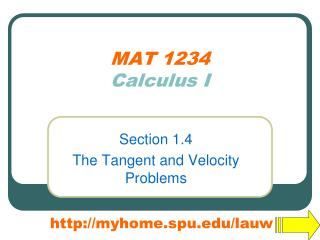DownloadDownload PresentationMAT 1234 Calculus I

# MAT 1234 Calculus I

Télécharger la présentation## MAT 1234 Calculus I

- - - - - - - - - - - - - - - - - - - - - - - - - - - E N D - - - - - - - - - - - - - - - - - - - - - - - - - - -
##### Presentation Transcript

1. MAT 1234Calculus I Section 1.4 The Tangent and Velocity Problems http://myhome.spu.edu/lauw

2. WebAssign • Homework 1.4 • Good News…Only 2 problems… • If you need help on the first assignment that due tomorrow, tutoring is open after class! • Happy Friday!

3. The Winner of the Contest is…

4. Preview • Big Picture: Why do we study differential calculus this quarter?

5. What do we care? • How fast “things” are going • The velocity of a particle • The “speed” of formation of chemicals • The rate of change of a population

6. What is “Rate of Change”?

7. What is “Rate of Change”?

8. What is “Rate of Change”?

9. What is “Rate of Change”?

10. What is “Rate of Change”?

11. What is “Rate of Change”?

12. What is “Rate of Change”?

13. What is “Rate of Change”?

14. Tesla Model S on Fire • Tesla Stock Plummets After Model S Catches On Fire

15. What is “Rate of Change”?

16. What is “Rate of Change”?

17. What is “Rate of Change”?

18. What is “Rate of Change”?

19. What is “Rate of Change”?

20. What is “Rate of Change”? We are going to look at how to understand and how to find the “rate of change” in terms of functions.

21. The Problems • The Tangent Problem • The Velocity Problem

22. Example 1 The Tangent Problem We are going to use a “limiting” process to “find” the slope of the tangent line at x=1. Slope=?

23. Example 1 The Tangent Problem First we compute the slope of the secant line between x=1 and x=3. Slope=?

24. Example 1 The Tangent Problem Then we compute the slope of the secant line between x=1 and x=2. Slope=?

25. Example 1 The Tangent Problem As the point on the right hand side of x=1 getting closer and closer to x=1, the slope of the secant line is getting closer and closer to the slope of the tangent line at x=1. Slope=?

26. Example 1 The Tangent Problem First we compute the slope of the secant line between x=1 and x=3. Slope=?

27. Example 1 The Tangent Problem We want a formula to compute this over and over again with other points in terms of their distance. Observation….

28. Observation… Let h be the distance between the two points.

29. Example 1 The Tangent Problem Let us record the results in a table.

30. Example 1 The Tangent Problem We “see” from the table that the slope of the tangent line at x=1 should be _________.

31. Limit Notations When h is approaching 0, is approaching 1. We say as h0, Or,

32. Definition For the graph of , the slope of the tangent line at is if it exists.

33. Two Worlds and Two Problems

34. Example 2 The Velocity Problem y = distance dropped (ft) t = time (s) Displacement Function (Positive Downward) Find the velocity of the ball at t=2.

35. Example 2 The Velocity Problem Again, we are going to use the same limiting process. Find the average velocity of the ball from t=2 to t=2+h by the formula

36. Example 2 The Velocity Problem

37. Example 2 The Velocity Problem We “see” from the table that velocity of the ball at t=2 should be _________ft/s.

38. Example 2 The Velocity Problem We “see” from the table that velocity of the ball at t=2 should be 64ft/s. The instantaneous velocity at t=2 is 64 ft/s. (The ball is traveling at 64 ft/s 2 seconds after it dropped.)

39. Limit Notations When h is approaching 0, is approaching 64. We say as h0, Or,

40. Definition For the displacement function, the instantaneous velocity at is if it exists.

41. Two Worlds and Two Problems

42. Review and Preview • Example 1 and 2 show that in order to solve the tangent and velocity problems we must be able to find limits. • In the next few sections, we will study the methods of computing limits without guessing from tables.

43. Review and Preview • Example 1 and 2 show that in order to solve the tangent and velocity problems we must be able to find limits. • In the next few sections, we will study the methods of computing limits without guessing from tables. • (BTW, why guessing is not enough?)